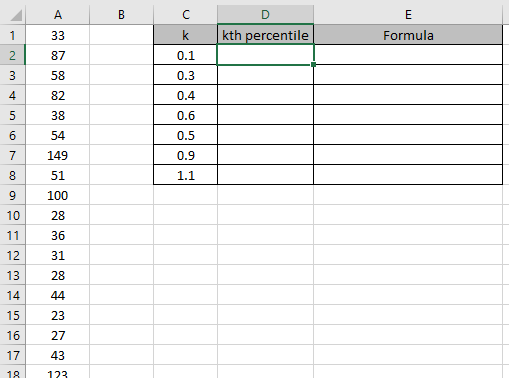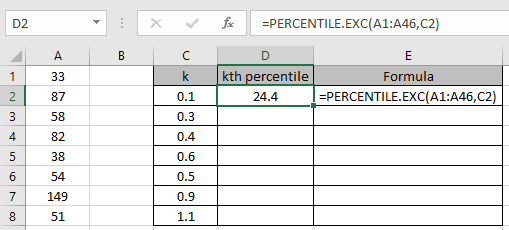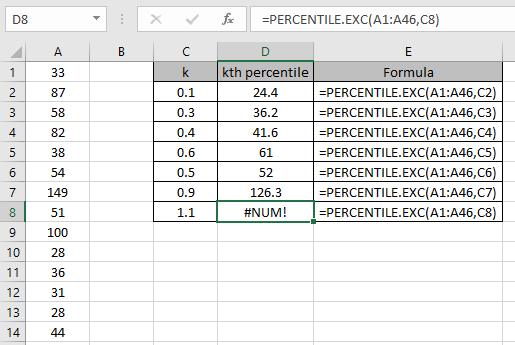# How to use the Excel PERCENTILE.EXC Function

The PERCENTILE.EXC is a statistical function. In statistics, finding a value below the given kth percentage or Percentile. Value of k can be shown in the below table.

 Value of k Percentage Percentile 0.1 10 % 10th percentile 0.2 20 % 20th percentile 0.3 30 % 30th percentile 0.4 40 % 40th percentile 0.5 50 % 50th percentile 0.6 60 % 60th percentile 0.7 70 % 70th percentile 0.8 80% 80th percentile 0.9 90% 90th percentile

PERCENTILE.EXC function takes the range of values as array and value of k. And returns the interpolate value from the array.
Syntax:

=PERCENTILE.EXC (array, k)

k : number between  0 & 1 . Excluding 0 & 1 itself.

Let’s understand this function using it as an example.

Here we have a set of values as A1 : A46 and we need to find some PERCENTILE.EXC values from the array.Use the formula:

= PERCENTILE.EXC ( A1 : A46 , C2)

A1 : A46 - array given as cell reference.
C2 - value of k given as cell reference.The function returns the value for K = 0.1 or 10th PERCENTILE value which is 24.4 .
Now copy the formula to get different PERCENTILE.EXC values of the range to the remaining cells and formula as shown in the snapshot below.As you can see the outcomes and the Formulas in the snapshot above.

Here are some of the observations gathered using the PERCENTILE.EXC function.

Note:

1. The function returns #VALUE! Error if the argument to the function is non numeric.
2. The function returns #NUM! error if the value of k is less than or equal to zero or greater than or equal to 1.
3. The array argument to the function ignores text, Logic values or Date & Time values.

Hope you understood How to use PERCENTILE.EXC function in Excel. Explore more articles on statistical function like PERCENTILE function & PERCENTILE.INC function here. Please state your query in the comment box below.

Related Articles

How to use the PERCENTILE.EXC function in Excel

How to use the LARGE function in Excel

How to use the SMALL function in Excel

How to use Wildcards in Excel

Popular Articles

Edit a dropdown list

If with conditional formatting

If with wildcards

Vlookup by date

Terms and Conditions of use

The applications/code on this site are distributed as is and without warranties or liability. In no event shall the owner of the copyrights, or the authors of the applications/code be liable for any loss of profit, any problems or any damage resulting from the use or evaluation of the applications/code.